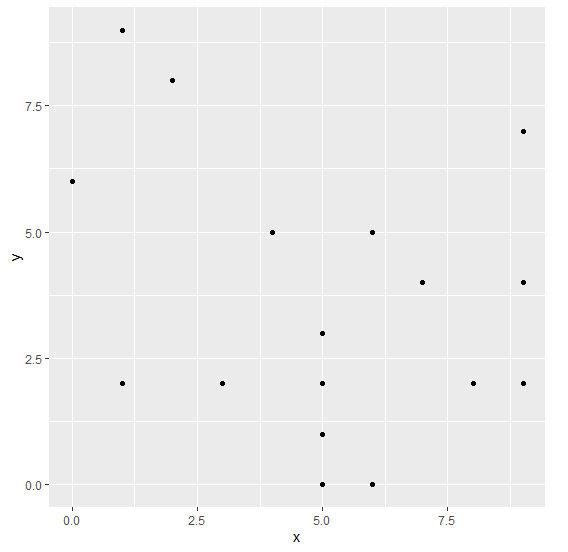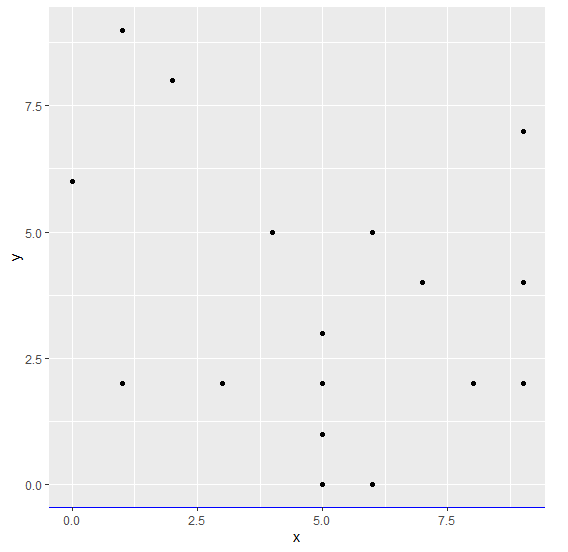# Change the color of X-axis line for a graph using ggplot2.

To change the color of X-axis line for a graph using ggplot2, we can use theme function where we can set the axis.line.x.bottom argument color to desired color with element_line.

Check out the below Example to understand how it can be done. This might be required when we want to highlight the X-axis for viewers.

## Example

Following snippet creates a sample data frame −

x<-sample(0:9,20,replace=TRUE)
y<-sample(0:9,20,replace=TRUE)
df<-data.frame(x,y)
df

The following dataframe is created

   x y
1  4 5
2  5 0
3  5 3
4  7 4
5  1 9
6  0 6
7  6 0
8  9 7
9  6 5
10 5 3
11 2 8
12 3 2
13 5 1
14 1 2
15 8 2
16 6 5
17 5 2
18 1 2
19 9 4
20 9 2

To create ggplot2 package and to create a scatterplot between x and y on the above created data frame, add the following code to the above snippet −

x<-sample(0:9,20,replace=TRUE)
y<-sample(0:9,20,replace=TRUE)
df<-data.frame(x,y)
library(ggplot2)
ggplot(df,aes(x,y))+geom_point()


## Output

If you execute all the above given snippets as a single program, it generates the following Output −To create a scatterplot between x and y with blue colored X-axis line on the above created data frame, add the following code to the above snippet −

x<-sample(0:9,20,replace=TRUE)
y<-sample(0:9,20,replace=TRUE)
df<-data.frame(x,y)
library(ggplot2)
ggplot(df,aes(x,y))+geom_point()+theme(axis.line.x.bottom=element_line(color="blue"))


## Output

If you execute all the above given snippets as a single program, it generates the following Output −